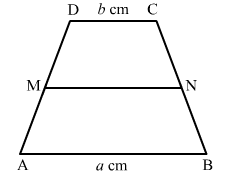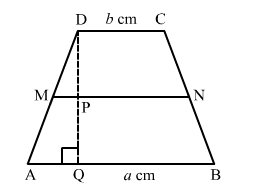# In a trapezium ABCD, AB || DC, AB = a cm, and DC = b cm. If M and N are the midpoints of the nonparallel sides,

Question:

In a trapezium ABCDAB || DC, AB a cm, and DC b cm. If and N are the midpoints of the nonparallel sides, AD and BC respectively then find the ratio of ar(DCNM) and ar(MNBA).Solution:Construction: Draw a perpendicular from point D to the opposite side AB, meeting AB at Q and MN at P.
Let length DQ = h
Given, M and N are the midpoints of AD and BC respectively.

So, $\mathrm{MN}\|\mathrm{AB}\| \mathrm{DC}$ and $\mathrm{MN}=\frac{1}{2}(\mathrm{AB}+\mathrm{DC})=\left(\frac{a+b}{2}\right)$

M is the mid point of AD and MP || AB so by converse of mid point theorem,
MP || AQ and P will be the mid point of DQ.

$\operatorname{ar}(\mathrm{DCNM})=\frac{1}{2} \times \mathrm{DP}(\mathrm{DC}+\mathrm{MN})=\frac{1}{2} h\left(b+\frac{a+b}{2}\right)=\frac{h}{4}(a+3 b)$

$\operatorname{ar}(\mathrm{MNBA})=\frac{1}{2} \times \mathrm{PQ}(\mathrm{AB}+\mathrm{MN})=\frac{1}{2} h\left(a+\frac{a+b}{2}\right)=\frac{h}{4}(b+3 a)$

ar(DCNM) : ar(MNBA) = (a + 3b) : (3a + b)